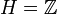# Subgroup of abelian group not implies powering-invariant

This article gives the statement and possibly, proof, of a non-implication relation between two subgroup properties. That is, it states that every subgroup satisfying the first subgroup property (i.e., subgroup of abelian group) need not satisfy the second subgroup property (i.e., powering-invariant subgroup)
View a complete list of subgroup property non-implications | View a complete list of subgroup property implications
Get more facts about subgroup of abelian group|Get more facts about powering-invariant subgroup
EXPLORE EXAMPLES YOURSELF: View examples of subgroups satisfying property subgroup of abelian group but not powering-invariant subgroup|View examples of subgroups satisfying property subgroup of abelian group and powering-invariant subgroup

## Statement

It is possible to have an abelian group$G$ and a subgroup$H$ of$G$ that is not a powering-invariant subgroup of$G$: there exists a prime number$p$ such that$G$ is powered over$p$, but$H$ is not powered over$p$.

## Related facts

Thus, our example must be an infinite subgroup of infinite index.

## Proof

The simplest example is as follows:$G = \mathbb{Q}$ is the additive group of rational numbers and$H = \mathbb{Z}$. In this case,$G$ is powered over all primes, but$H$ is not powered over any.